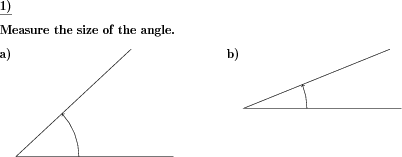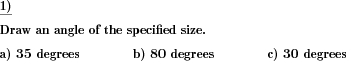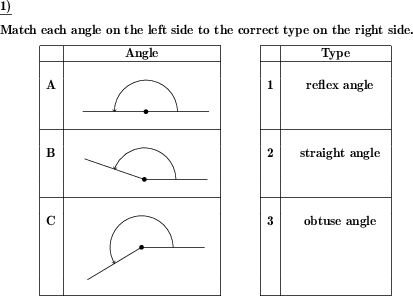Custom math worksheets at your fingertips# Details for problem "Angle size measurement"

Quickname: 4788

Elementary School, Primary School, Junior High School, Middle School, High School.

## Summary

Measure the size of a given angle

## Example## Description

Angles are one of the basic concepts in geometry and hence it is a requisite to understand the measurement of angles. The size of a given angle has to be measured that is the turn from one arm of the angle to the other.

The problem is to be used as small group work, remediation, quiz, test, drills, unit assessment tool helps to achieve mastery in learning angle measurement. It will provide a possibility to achieve other milestones mentioned below.

1. Recalling the concept of measurement.
2. Learn the use of a protractor to measure a certain angle.
3. Understand the minimum and maximum angles.
4. Relate fractions, angles, and degrees.
5. Estimate the measure of the angle.

• The number of problems can range from 1 to 10.
• The length of the rays can be selected from two sizes i.e, 5 cm and 8 cm.
• A minimum and a maximum size, from 0 to 360, can be specified.
• The size may be chosen to adhere to a five or ten-degree raster, and sizes will be multiples of this value.

The answer key will provide the measurement of angle in all problems.

Download free printable worksheets for this math problem here. The worksheet contains the problems only, the solution sheet includes the answers. Just click on the respective link.

•Worksheet 1Solution sheet with answers
•Worksheet 2Solution sheet with answers
•Worksheet 3Solution sheet with answers

If you can not see the solution sheets for download, they may be filtered out by an ad blocker that you may have installed. If this is the case, please allow ads for this page and reload the page. The solution sheets will then reappear.

These worksheets contain math problems that have to be printed with the correct aspect ratio. When printing, please take care to switch all automatic adjustments, scaling of page, auto adjust, etc, off.

• Do these sample worksheets do not really fit?
• Do you need more math worksheets, with a different level of difficulty?
• Would you like to combine different problems on a worksheet and adjust them to your needs?
• As a teacher, you can put together your own worksheets using the automatically generated math problems provided.
With a free initial credit, you can start creating your own math worksheets in a few minutes.

You can try it for free! Register here, to create custom worksheets now!

## Customization options for this problem

Parameter
Possible values
Number of problems
1, 2, 3, 4, 5, 6, 7, 8, 9, 10
Min degrees
Numeric value from 0 to 360.
Max degrees
Numeric value from 0 to 360.
Raster
None, 5 degrees, 10 degrees
Ray length
5 cm, 8 cm

## Similar problems

Remark
Description
For a given angle, the correct type has to be marked.

## dw-Math worksheet templates that do contain this problem

Name
Title
Description
Angles and angle types
Measure and draw angles, identify types of angles

## Other types of problems that appear on worksheets with this problem:

Relevance
Name
Description
Quickname
Example
****
Angle types
For a given angle, the correct type has to be marked.****
Angle to be drawn
An angle of a given size has to be drawn.**
Match angles to angle types
Given angles have to be matched to given typesDeutsche Version dieser Aufgabe
These informational pages with samples describe math problems that can be combined on custom math worksheets with solutions for home and K-12 school use.
Deutsche Seiten
×